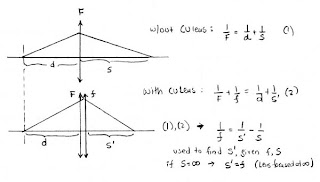## Saturday, February 9, 2008

### Macro Photography with Close-Up LensesWhen extension is not an option (because the lens is fixed to the camera body, for example) an alternative way to focus closer is to use close-up (or supplementary) lenses.

Close up lenses are marked in diopters. A diopter is 1/F, where F is the focal length of the close-up lens expressed in meters. So a +1 lens has a focal length of 1 meter (40 inches), a +2 lens has a focal length of 0.5 meters (20 inches) etc.

Assume that the lens of focal length F is focused on an object at distance s. The image is formed at a distance d behind the lens. From the fundamental lens equation we get: 1/F = 1/s + 1/d. (1)

Now, let’s insert a close-up lens with focal length f, in front of this lens. Without touching the focus of our lens (so d does not change), now we have to place our object closer to the lens, at a distance s’, in order to have it in focus. The combined strength of the two lenses now is F + f, and the fundamental lens formula now becomes: 1/F + 1/f = 1/d + 1/s; (2)

Subtracting (1) from (2) we get: 1/f = 1/s' – 1/s, or 1/s' = 1/s + 1/f (3)

This formula can be used to calculate the sharp focus distance (s’) when using a supplementary lens (f), while our original lens is focused at distance (s). What is interesting is that the focal length of the original lens is not involved. So, we can construct tables that universally apply for all lenses. Another interesting observation is that if our original lens is focused at infinity (1/s = 0) then the sharp focus distance is equal to the focal length of the supplementary lens.

Based on (3) we can construct this useful table for lens whose close focus is 1m (about 3 ft, common close focus distance in many lenses):

Close Up Lens / Infinity Focus / Close Focus
--------------------------------------------
None infinity 1m
+1 1m 0.5m
+2 0.5m 0.33m
+4 0.25m 0.20m

Two more comments: 1) Close-up lenses can be stacked. In this case, their powers are added. If we stack a +1 and +2 lens, we get a combined strength of +3. 2) It is recommended that the aperture of the lens is kept closed (f8 or smaller) when using supplementary lenses, both to get a decent depth of field and also to keep the aberrations down.

When using close-up lenses with rangefinder cameras, a focal frame is useful for framing the subject. An example is shown in the picture here. This is taken from Rodolf Kingslake’s book “Optics in Photography”. I highly recommend this book for anyone interested in this topic.